International Olympiad of Mathematics (iOM) is conducted by Silver Zone Foundation annually at National and International levels for the students of classes 1 to 12. It aims at testing students mathematical skill and knowledge of a student based on CBSE or other state board syllabus. iOM is a 3 level exam. The last date to register for the exam is September 30, 2019.

• iOM level 1 will be held on November 13 and December 13, 2019.
• The result for level 1 will be declared in the fourth week of January 2020. Students qualifying level 1 will appear for iOM level 2 which is conducted at the national level.
• The exam for level 2 will be held in candidate's state or city while the level 3 exam will be conducted at New Delhi.
• Result for level 2 will be released in the third week of March 2020. Students who qualify level 3 and hold rank 1 & 2 are sent to NASA for an educational excursion.
• Other students will also receive participation certificates, cash prizes and medals to toppers at each level.
 Exam level: Intermediate Frequency: Once a year Languages English Conducting Body: SilverZone Foundation Duration 1 Hour

iOM 2019 Important Dates

Upcoming Dates and Events

13 Nov, 2019

Exam | Mode: Offline

13 Dec, 2019

Exam | Mode: Offline

Past Dates and Events

01 Jun, 2019 - 30 Sep, 2019

Application | Mode: Offline

iOM 2019 Eligibility Criteria

iOM eligibility criteria state that -

• iOM level 1 allows all students from classes 1-12 to participate in the exam.
• For level 2, students holding top 1000 rank from each class in level 1 are eligible to appear.
• Only rank 1 holders from class 6 to 12 in level 2 are eligible to appear for the level 3 exam.

iOM 2019 Application Process

Mode of Application : Offline

Mode of Payment : Other

The application process for iOM level 1 is held through schools. The last date to register is September 30, 2019. The students needs to register themselves with corresponding schools. No, students are allowed for individual registration.

Application Fees

Category Quota Mode Gender Amount
ST, General, SC, OBC Offline Male, Transgender, Female ₹ 120

iOM 2019 Syllabus

IOM class 1
Skip counting
Back and forth counting
Ones
Tens and hundreds
Subtraction
Comparison
Time and calendar
Money
Identification of geometrical figures
Number series
Measurement
Knowing patterns
Applied mathematics
Mental aptitude
IOM class 2
Ones
Tens
Hundreds and thousands
Subtraction
Multiplication
Division
Comparison
Time and calendar
Money
Geometrical figures
Measurement
Number series
Knowing patterns
Applied mathematics
Mental aptitude
IOM class 3
Number sense and numeration
Operations on numbers
Fractions
Time and calendar
Money
Unitary method
Measurement (length, mass, capacity)
Geometrical shapes
Data handling
Pictograph and bar graph
Reasoning and aptitude
IOM class 4
Number sense and numeration
Numbers and their operations
Roman numerals
Factors and multiples
Fractions and decimals
Money
Unitary method
Geometrical shapes
Perimeter and area of geometrical shapes
Data handling
Graphical representation of data
Reasoning and aptitude
IOM class 5
Number sense and numeration
Numbers in operations
Roman numerals
Factors and multiples
Fractions and decimals
Percentage
Ratio and proportion
Time and distance
Average
Algebra
Measurement (perimeter, area, volume, time, temperature)
Geometry
Data handling
Graphical representation of data
Reasoning and aptitude
IOM class 6
Numbers and their operations
Factors and multiples
Fractions and decimals
Ratio and proportion
Algebra
Geometry and symmetry
Circle
Mensuration (perimeter, area and volume)
Data handling
Graphical representation of data
Sets
Reasoning and aptitude
IOM class 7
Number system and operations
Fractions and decimals
Rational numbers
Exponents and powers
Algebraic expressions
Linear equations
Ratio and proportion
Percentage
Profit and loss
Annual instalment
Simple interest and compound interest
Lines
Angles
Triangles and circle
Mensuration
Data handling
Sets
Reasoning and aptitude
IOM class 8
Number systems
Powers and exponents
Square and square root
Cube and cube root
Algebraic expressions
Factorization
Linear equation
Direct and inverse proportions
Time and work
Percentage
Profit and loss
Compound interest and simple interest
Geometry
Mensuration
Data handling
Applied mathematics
Reasoning and aptitude
IOM class 9
Real numbers
Polynomials
Logarithms
Linear equation in two variables
Line and angles
Triangles
Area of triangles
Parallelograms and circles
Trigonometric ratios and identities
Mensuration
Statistics
Probability
Coordinate geometry
Word problems based on mathematics
Verbal and non-verbal reasoning
IOM class 10
Number system
Polynomials
Linear equation
Arithmetic progression
Coordinate geometry
Statistics
Trigonometry
Height and distance
Circles
Triangles
Probability
Sequence and series
Mensuration
Verbal and non-verbal reasoning
IOM class 11
Sets and relation and function
Trigonometric functions
Complex number
Sequence and series
Permutation and combination
Binomial theorem
Coordinate geometry
Limits and derivatives
Statistics
3D geometry
Vectors
Probability
Logarithm
Inequality
Applied mathematics
Mental aptitude
IOM class 12
Relation and function
Inverse trigonometric function
Matrices and determinants
Limits and continuity
Differentiation
Application of derivatives
Integration
Application of integral
Differential equation
Vectors
3D
Linear programming
Probability
Applied mathematics
Mathematical reasoning
Mental aptitude

iOM 2019 Preparation Tips

ddddd

How to prepare for iOM 2019 exam?

• Students must follow the prescribed syllabus for iOM.
• Practice iOM sample papers as many as possible to have a better idea about exam pattern, marking scheme, no. of questions etc.
• Revise regularly and follow a proper schedule to successfully crack the iOM 2019 exam.
• Eat healthy food and have proper rest, exercise or play games in between to refresh your mind and body.

iOM 2019 Exam Pattern

According to the exam pattern of iOM, it is a multiple choice paper for 1 hour with no negative marking. It comprises 40 questions for class 1 to 2 and 50 questions for class 3 to 12. The detailed class wise iOM exam pattern is given below:

Class 1 and 2

 Unit No. of questions Marks Total             marks Counting             numbers 3 2.5 7.5 Operation on numbers 10 2.5 25 Time and calendar 2 2.5 5 Money 1 2.5 2.5 Measurement 3 2.5 7.5 Geometrical shapes 5 2.5 12.5 Reasoning and Aptitude 8 2 16 Scholar’s zone 8 3 24 Total question 40 Total             marks 100

Class 3

 Unit No.  of questions Marks Total             marks Numbers and their Operations 11 2 22 Fractions 4 2 8 Everyday             Mathematics 3 2 6 Measurement 4 2 8 Geometrical             Shapes 5 2 10 Data Handling 3 2 6 Reasoning and Aptitude 10 1.5 15 Scholar’s zone 10 2.5 25 Total question 50 Total             marks 100

Class 4

 Unit No. of questions Marks Total             marks Numbers and their Operations 10 2 20 Fractions and Decimals 5 2 10 Everyday Mathematics 2 2 4 Geometrical Shapes 5 2 10 Perimeter and Area 5 2 10 Data handling 3 2 6 Reasoning and Aptitude 10 1.5 15 Scholar’s zone 10 2.5 25 Total question 50 Total             marks 100

Class 5

 Unit No. of questions Marks Total             marks Numbers and there Operations 11 2 22 Fractions and Decimals 4 2 8 Everyday Mathematics 4 2 8 Geometry and Measurement 7 2 14 Data Handling 3 2 6 Algebra 1 2 2 Reasoning and Aptitude 10 1.5 15 Scholar’s zone 10 2.5 25 Total question 50 Total             marks 100

Class 6

 Unit No. of questions Marks Total             marks Numbers and their Operations 9 2 18 Fractions and Decimals 5 2 10 Algebra, Ratio and Proportion 5 2 10 Geometry and Measurement 7 2 14 Data Handling 3 2 6 Sets 1 2 2 Reasoning and Aptitude 10 1.5 15 Scholar’s zone 10 2.5 25 Total question 50 Total             marks 100

Class 7

 Unit No. of questions Marks Total             marks Numbers and their Operations 12 2 24 Algebra 3 2 6 Everyday Mathematics 4 2 8 Geometry and Measurement 7 2 14 Data Handling 3 2 6 Sets 1 2 2 Reasoning and Aptitude 10 1.5 15 Scholar’s zone 10 2.5 25 Total question 50 Total             marks 100

Class 8

 Unit No. of questions Marks Total             marks Number Systems and Operations 6 2 12 Algebra and Geometry 6 2 12 Comparing Quantities 8 2 16 Mensuration 4 2 8 Data Handling 4 2 8 Applied Mathematics 2 2 4 Reasoning and Aptitude 10 1.5 15 Scholar’s zone 10 2.5 25 Total question 50 Total            marks 100

Class 9

 Unit No. of questions Marks Total             marks Real Numbers 4 2 8 Algebra and Co-ordinate Geometry 8 2 16 Geometry 5 2 10 Trigonometry 3 2 6 Statistics and Probability 5 2 10 Applied Mathematics 5 2 10 Reasoning and Aptitude 10 1.5 15 Scholar’s zone 10 2.5 25 Total question 50 Total            marks 100

Class 10

 Unit No. of questions Marks Total       marks Number System & Sequence and Series 5 2 10 Algebra and Co-ordinate Geometry 5 2 10 Geometry 5 2 10 Trigonometry 5 2 10 Statistics and Probability 5 2 10 Mensuration 5 2 10 Reasoning and Aptitude 10 1.5 15 Scholar’s zone 10 2.5 25 Total question 50 Total            marks 100

Class 11

 Unit No. of questions Marks Total             marks Sets & Functions 8 2 16 Algebra 8 2 16 Co-ordinate Geometry and Vectors 6 2 12 Calculus 3 2 6 Mathematical Reasoning 1 2 2 Statistics and Probability 4 2 8 Reasoning and Aptitude 10 1.5 15 Scholar’s zone 10 2.5 25 Total question 50 Total             marks 100

Class 12

 Unit No.             of questions Marks Total             marks Relations and Functions 5 2 10 Algebra 4 2 8 Vectors & 3D 6 2 12 Calculus 10 2 20 Linear Programming and Mathematical Reasoning 1 2 2 Probability 4 2 8 Reasoning and Aptitude 10 1.5 15 Scholar’s zone 10 2.5 25 Total question 50 Total             marks 100

iOM 2019 Result

• Level 1 result will be announced on January 2020 in online mode.
• Qualified candidates of level 1 appeared for level 2. The result of level 2 will be declared in March 2020.
• Level 3 exam will be conducted in the month of May/June 2020 and result for same will be released in the month of August/September 2020.

Related Exams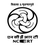National Talent Search Examination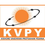Kishore Vaigyanik Protsahan Yojana Exami ...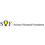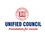National Level Science Talent Search Exa ...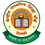Central Board of Secondary Education Cla ...
View All Related Exams

News and Articles

View More
The question have been saved in answer later, you can access it from your profile anytime. Access now